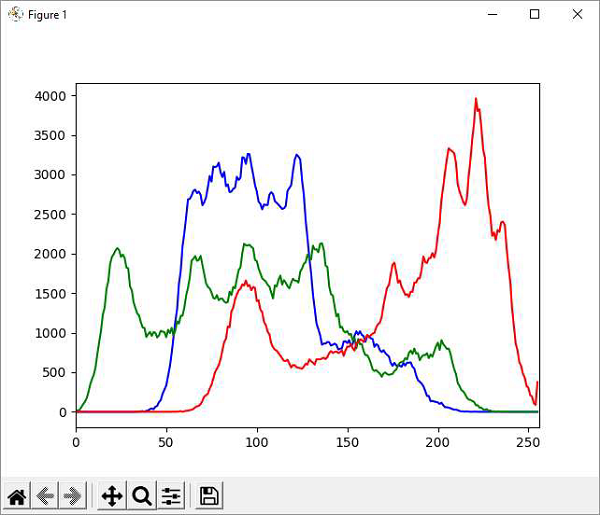# OpenCV Python - Histogram

Histogram shows the intensity distribution in an image. It plots the pixel values (0 to 255) on X axis and number of pixels on Y axis.

By using histogram, one can understand the contrast, brightness and intensity distribution of the specified image. The bins in a histogram represent incremental parts of the values on X axis.

In our case, it is the pixel value and the default bin size is one.

In OpenCV library, the function cv2.calcHist() function which computes the histogram from the input image. The command for the function is as follows −

cv.calcHist(images, channels, mask, histSize, ranges)


## Parameters

The cv2.calcHist() function’s parameters are as follows −

• images − It is the source image of type uint8 or float32, in square brackets, i.e., "[img]".

• channels − It is the index of the channel for which we calculate histogram. For a grayscale image, its value is . For BGR images, you can pass ,  or  to calculate the histogram of each channel.

• mask − Mask image is given as "None" for full image. For a particular region of image, you have to create a mask image for that and give it as a mask.

• histSize − This represents our BIN count.

• ranges − Normally, it is [0,256].

## Histogram using Matplotlib

A histogram plot can be obtained either with the help of Matplotlib’s pyplot.plot() function or by calling Polylines() function from OpenCV library.

## Example

Following program computes histogram for each channel in the image (lena.jpg) and plots the intensity distribution for each channel −

import numpy as np
import cv2 as cv
from matplotlib import pyplot as plt